# Hydrothermal Behavior of Transient Fluid Flow and Heat Transfer Through a Rotating Curved Rectangular Duct with Natural and Forced Convection

Hydrothermal Behavior of Transient Fluid Flow and Heat Transfer Through a Rotating Curved Rectangular Duct with Natural and Forced Convection

Ratan Kumar Chanda Mohammad Sanjeed Hasan Md. Mahmud Alam Rabindra Nath Mondal

Department of Mathematics, Jagannath University, Dhaka 1100, Bangladesh

Department of Mathematics, Bangabandhu Sheikh Mujibur Rahman Science and Technology University, Gopalganj 8100, Bangladesh

Mathematics Discipline, Khulna University, Khulna 9208, Bangladesh

Corresponding Author Email:
rnmondal@jnu.ac.bd
Page:
501-514
|
DOI:
https://doi.org/10.18280/mmep.070401
20 July 2020
|
Accepted:
15 December 2020
|
Published:
18 December 2020
| Citation

OPEN ACCESS

Abstract:

The present work explores a spectral-based computational study on hydrothermal behavior of transient fluid flow with natural and forced convective heat transfer through a rotating curved rectangular duct of strong curvature. The outer wall of the duct is heated while the inner wall cooled, the other walls being thermally insulated. The system rotates about the vertical axis in the positive and negative direction for the Taylor number (-1000≤Tr≤1000) with a constant non-dimensional pressure gradient force, the Dean number Dn = 2000. Time-history analysis is performed and fluid characteristics are well determined by depicting the phase space of the time-history result. It is found that the chaotic flow turns into steady-state flow through multi-periodic and periodic oscillating flows, if Tr is increased either in the positive or in the negative direction. Streamlines of secondary flow and isotherms are obtained at some specific values of Tr, and it is found that the time-dependent flow consists of asymmetric 2- to multi-vortex solutions. Vortex structure of secondary flows is obtained for physically realizable solutions and it is found that maximum 8-vortex is obtained for the chaotic solution while 2-vortex for the steady-state solution. Nusselt number as well as temperature gradient is calculated as an index of heat transfer, and it is found that convective heat transfer is significantly enhanced by the secondary flow; and the chaotic flow, which occurs relatively at small Tr, boosts heat transfer more effectively than the steady-state or other solutions. Finally, our numerical results have been validated with the experimental outcomes and it is found that there is a good agreement between the numerical and experimental investigations.

Keywords:

rotating curved duct, Taylor number, secondary flow, isotherm, time-progression

1. Introduction

Due to ample applications from medical services to industrial activities, the study of flow and heat transfer through a curved duct has attracted considerable attention to the researchers from the past to the present. When a fluid flows in a curved duct, the flow experiences helical streamlines. This is called secondary flow, which is a relatively minor flow imposed on the primary flow. The secondary flow is found in numerous flow situations such as in the blade passage in turbo-machinery, a bent pipe, internal combustion engines, aircraft intakes, refrigeration, air conditioning systems, diffusers and heat exchangers, not to mention applications in other areas such as blood flow in human and other animals. The magnitude of these secondary flows is quantified by a non-dimensional parameter, the Dean number, Dn, defined by, $D n=R e \sqrt{\frac{D_{h}}{2 R}}$, where Re is the Reynolds number, Dh is the hydraulic diameter and R is the radius of curvature of the curved channel. When fluid is flowing through a curved channel, centrifugal force takes into effect and the flow velocity closer to the outer wall is greater than the flow velocity at the inner wall. As the fluid near the outer wall moves with relatively large velocity, an apparent vacuum at the inner wall is generated immediately in front of the flow and the fluid closer to the outer wall shows a tendency to move towards the inner wall. This circulatory flow from the outer curved wall to the inner wall is named as Dean vortices in recognition of the pioneer work by Dean . After that, a significant number of theoretical and experimental investigations have been made; here the articles by Berger et al. , Nandakumar and Masliyah , Ito , Yanase et al.  and Mondal  may be referenced.

It is worth mentioning that the fluid flowing in a rotating curved duct is subjected to two forces: the Coriolis force and the centrifugal force. Due to combined action of these two forces a complex behavior of the secondary flow and the axial flow is obtained, and when a temperature induced variation of fluid density occurs for non-isothermal flows, both Coriolis and centrifugal forces contribute to the generation of vorticity . Ghia and Shokhey  showed that the duct aspect ratio and curvature ratio significantly influence Dean instability in the curved channel. Ishigaki  examined the flow structure and friction factor for both counter-rotating and co-rotating curved pipe with small curvature. Selmi et al.  examined combined effects of system rotation and curvature on the bifurcation structure of two-dimensional flows in a rotating curved square duct. Wang and Cheng  examined the flow characteristics and heat transfer in curved square ducts for positive rotation and found reverse secondary flow for the co-rotation cases. Recently, Mondal et al.  predicted a numerical study on curved rectangular duct flow and compared their results with the experimental data obtained by Chandratilleke et al.  and visualized the stream functions and the isotherms for different Dean numbers and the Grashof number. Norouzi et al.  elaborated first and second normal stresses with enumerating the Nusselt number and the influence on secondary flow intensity in the elastic property. For curved rectangular duct, Chandratilleke et al.  reported an extensive parametric study, capturing the profound influence from duct aspect ratio, curvature ratio and wall heat flux on the flow behavior. Recently, Hasan et al.  and Hasan et al.  employed spectral method to study steady solutions and unsteady flow characteristics through a rotating and non-rotating curved square duct for constant curvature, and showed combined effects of centrifugal-Coriolis-buoyancy forces on fluid flows in the curved channel.

With a view to study non-linear behavior of the unsteady solutions, Yanase and Nishiyama  first initiated time-dependent solutions through a curved rectangular duct. Wang and Yang  performed numerical as well as experimental investigation on fully developed periodic oscillation in a curved square duct. Mondal et al.  performed numerical prediction of isothermal flow with unsteady solutions through a stationary curved square duct, where they showed that periodic solution turns into chaotic solution via multi-periodic solution, if the Dean number is increased. The secondary flow characteristics in a curved square duct were investigated experimentally by using visualization method by Yamamoto et al. . Mondal et al.  performed numerical prediction of non-isothermal flow with unsteady solutions through a stationary curved square duct, where they showed that periodic solutions turn into chaos through a multi-periodic solution, if the Dean number is increased no matter what the curvature is. Time evolution calculation with the variation of centrifugal and Coriolis instability has been predicted by Mondal et al.  at a high-pressure gradient over a wide range of the Taylor number. Unsteady flow characteristics, heat transfer and entropy generation has been performed by Razavi et al. . For solving the unsteady flow through a curved tube with strong curvature, recently Krishna et al.  performed flow visualization and computational fluid dynamics. Recently, Islam et al.  showed transitional behavior of the flow in a rotating curved duct for various Dean numbers over a wide range of the Taylor number. Very recently, Hasan et al. , Hasan et al.  and Hasan et al.  studied unsteady behavior of the flow through a rotating curved square duct for constant Dean number. However, hydro-thermal behavior of the unsteady solution is not yet resolved, in detail, for the flow through a rotating curved rectangular duct with strong curvature to predict the influence of centrifugal and Coriolis forces on fluid flows, which motivated us to do this study to fill up this gap.

To study heat transfer through curved channels, Chandratilleke  reported experimental results for the flow in curved rectangular duct with external heating of the outer wall. He showed that convective heat transfer is significantly enhanced by the secondary flow particularly when the Dean vortices emerge at the outer wall. Yanase et al.  and Mondal et al.  studied time-dependent behavior of the unsteady solutions for curved rectangular/square duct flow and showed that secondary flows enhance heat transfer in the flow. Norouzi et al.  investigated fully developed flow and heat transfer of viscoelastic materials in curved square ducts under constant heat flux. Chandratilleke et al.  presented extensive 3D computational study using helicity function that describes the secondary vortex structure and thermal behavior in the fluid flow through curved rectangular ducts of aspect ratios ranging from 1 to 6. Norouzi and Biglari  performed, for the first time, an analytical solution of Dean flow inside a curved rectangular duct by using perturbation method. Their study was limited to low Reynolds numbers and obtained maximum four-vortex solutions. Wu et al.  performed numerical study of the secondary flow characteristics in a curved square duct by using spectral method, where the walls of the duct except the outer wall rotate around the centre of curvature and an azimuthal pressure gradient was imposed. Recently, Hasan et al. [35, 36] undertook numerical prediction of fluid flow and heat transfer through a non-rotating curved square channel for various Dean and Grashof numbers. The study elucidated role of secondary vortices on convective heat transfer which showed that convective heat transfer is significantly enhanced by the secondary flow; and the chaotic flow, which takes place at large Dean and Grashof numbers, enhances heat transfer more efficiently. Very recently, Ray et al.  applied spectral method to predict hydrodynamic instability and convective heat transfer through a rotating curved rectangular duct of moderate curvature. They obtained three branches of steady solutions and showed that chaotic flow turns into steady-state flow through periodic oscillating flow, if Tr is increased in the positive direction. As par to the authors' knowledge, however, there has not been done any substantial work studying the hydro-thermal behavior of fluid flow with forced and natural convective heat transfer through a rotating curved rectangular channel in the presence of strong centrifugal force for both positive and negative rotation of the channel. But from the scientific as well as engineering point of view it is quite interesting to study this type of flow because it is often encountered in engineering applications such as in gas turbine, plastic industry and metallic industry.

In the ongoing paper, our intention is to study hydro-thermal behavior with flow transition through a rotating curved rectangular duct of strong curvature. Studying the vortex structure as well as effects of rotation on the unsteady flow characteristics, caused by the combined action of the centrifugal, Coriolis and buoyancy forces, is an important objective of the present study.

2. Mathematical Model and Governing Equations

Consideration is given for hydro-dynamically and thermally fully developed two-dimensional laminar flow of viscous incompressible fluid streaming through a rotating curved rectangular duct of aspect ratio 2 and curvature ratio 0.5. The system rotates at a variable angular velocity $\Omega_{T}$ around the y' axis. It is assumed that the outer wall of the duct is heated while the inner wall cooled, the top and bottom walls being thermally insulated. The x-, y- and z-axes are taken to be in the horizontal, vertical and axial directions respectively. It is assumed that the flow is driven by a as shown in Figure 1.

## 1.jpgFigure 1. (a) Coordinate system, (b) Cross section of the curved duct

The dimensional variables are non-dimensionalized by using the representative length d and the representative velocity $U_{0}=\frac{v}{d}$, where υ is the kinematic viscosity of the fluid. The stream functions for cross-sectional velocities have the following form:

$\left.\begin{array}{l}u=\frac{1}{r} \frac{\partial \psi}{\partial y}=\frac{1}{1+\delta x} \frac{\partial \psi}{\partial y} \\ v=\frac{1}{r} \frac{\partial \psi}{\partial x}=-\frac{1}{1+\delta x} \frac{\partial \psi}{\partial x}\end{array}\right\}$  (1)

Now, stream-wise velocity (w), cross-sectional stream-function (ψ) and temperature profile (T) are derived based on the Navier-Stokes equation and energy equation as follows:

$(1+\delta x) \frac{\partial w}{\partial t}=D n-\frac{\partial(w, \psi)}{\partial(x, y)}+\frac{\delta^{2} w}{1+\delta x}+(1+\delta x) \Delta_{2} w-$$\frac{\delta}{1+\delta x} \frac{\partial \psi}{\partial y} w+\delta \frac{\partial w}{\partial x}-\delta \operatorname{Tr} \frac{\partial \psi}{\partial y} (2) \frac{\partial T}{\partial t}+\frac{1}{(1+\delta x)} \frac{\partial(T, \psi)}{\partial(x, y)}=\frac{1}{\operatorname{Pr}}\left(\Delta_{2} T+\frac{\delta}{1+\delta x} \frac{\partial T}{\partial x}\right) (3) \left(\Delta_{2}-\frac{\delta}{1+\delta x} \frac{\partial}{\partial x}\right) \frac{\partial \psi}{\partial t}=-\frac{1}{(1+\delta x)} \frac{\partial\left(\Delta_{2} \psi, \psi\right)}{\partial(x, y)}$$+\frac{\delta}{(1+\delta x)^{2}}\left[\frac{\partial \psi}{\partial y}\left(2 \Delta_{2} \psi-\frac{3 \delta}{1+\delta x} \frac{\partial \psi}{\partial x}+\frac{\partial^{2} \psi}{\partial x^{2}}\right)-\frac{\partial \psi}{\partial x} \frac{\partial^{2} \psi}{\partial x \partial y}\right]$

$+\frac{\delta}{(1+\delta x)^{2}} \times\left[3 \delta \frac{\partial^{2} \psi}{\partial x^{2}}-\frac{3 \delta^{2}}{1+\delta x} \frac{\partial \psi}{\partial x}\right]-\frac{2 \delta}{1+\delta x} \frac{\partial}{\partial x} \Delta_{2} \psi$$+w \frac{\partial w}{\partial y}+\Delta_{2}^{2} \psi-G r(1+\delta x) \frac{\partial T}{\partial x}+\frac{1}{2} \operatorname{Tr} \frac{\partial w}{\partial y}$   (4)

where,

$\Delta_{2} \equiv \frac{\partial^{2}}{\partial x^{2}}+\frac{1}{4} \frac{\partial^{2}}{\partial y^{2}}, \frac{\partial(f, g)}{\partial(x, y)} \equiv \frac{\partial f}{\partial x} \frac{\partial g}{\partial y}-\frac{\partial f}{\partial y} \frac{\partial g}{\partial x}$  (5)

In Eqns. (2)-(4), four non-dimensional parameters Dn, Gr, Pr and Tr are used which are defined as:

$D n=\frac{G d^{3}}{\mu v} \sqrt{\frac{2 d}{L}}, G r=\frac{\beta g \Delta T d^{3}}{v^{2}}, \operatorname{Pr}=\frac{v}{\kappa}, T r=\frac{2 \sqrt{2 \delta} \Omega d^{3}}{v \delta}$ (6)

The applied boundary conditions for w and ψ are used as:

$\left.\begin{array}{r}w(\pm 1, y)=w(x,\pm 1)=\psi(\pm 1, y)=\psi(x,\pm 1)=0 \\ \frac{\partial \psi}{\partial x}(\pm 1, y)=\frac{\partial \psi}{\partial y}(x,\pm 1)=0\end{array}\right\}$  (7)

and temperature T is assumed to be constant on the walls as:

$T(1, y)=1, \quad T(-1, y)=-1, \quad T(x,\pm 1)=x$  (8)

In the present study, Tr varies in both positive and negative, while Dn, Gr, δ and Pr are fixed as Dn = 2000, Gr = 100, δ=0.5 and Pr = 7.0 (water).

3. Numerical Computation

3.1 Procedure to numerical calculation

We applied spectral method to solve the Eqns. (2) to (4) numerically. By this method the variables are expanded in a series of functions consisting of Chebyshev polynomials. That is, the expansion functions $\varphi_{n}(x)$ and $\psi_{n}(x)$ are expressed as:

$\left.\begin{array}{l}\varphi_{n}(x)=\left(1-x^{2}\right) \quad C_{n}(x) \\ \psi_{n}(x)=\left(1-x^{2}\right)^{2} \quad C_{n}(x)\end{array}\right\}$  (9)

where, $C_{n}(x)=\cos \left(n \cos ^{-1}(x)\right)$ is the $n^{t h}$ order Chebyshev polynomial. $w(x, y, t), \psi(x, y, t)$ and T(x,y,t) are expanded in terms of the expansion functions $\varphi_{n}(x)$ and $\psi_{n}(x)$ as:

$\left.\begin{array}{rl}w(x, y, t) & =\sum_{m=0}^{M} \sum_{n=0}^{N} w_{m n}(t) \phi_{m}(x) \phi_{n}(y) \\ \psi(x, y, t) & =\sum_{m=0}^{M} \sum_{n=0}^{N} \psi_{m n}(t) \psi_{m}(x) \psi_{n}(y) \\ T(x, y, t) & =\sum_{m=0}^{M} \sum_{n=0}^{N} T_{m n}(t) \varphi_{m}(x) \varphi_{n}(y)+x\end{array}\right\}$  (10)

where, M  and N  are the truncation numbers in the x - and y -directions respectively, and $w_{m n}, \psi_{m n}$ and $T_{m n}$ are the coefficients of expansion. The collocation points $\left(x_{i}, y_{j}\right)$ are taken to be:

$\left.x_{i}=\cos \left[\pi\left(1-\frac{i}{M+2}\right)\right], y_{j}=\cos \left[\pi\left(1-\frac{j}{N+2}\right)\right]\right\}$  (11)

where, $i=1, \ldots, M+1$ and $j=1, \ldots, N+1$. In order to calculate the unsteady solutions, the Crank-Nicolson and Adams-Bashforth methods together with the function expansion (10) and the collocation methods are applied to Eqns. (2) to (4). Details of this method are available in Gottlieb and Orszag  and Mondal .

3.2 Resistance coefficient

The resistance coefficient λ is used as the representative quantity of the flow state. It is also called the hydraulic resistance coefficient, and is generally used in fluids engineering, defined as:

$\frac{P_{1}^{*}-P_{2}^{*}}{\Delta z^{*}}=\frac{\lambda}{d_{h}^{*}} \frac{1}{2} \rho\left\langle w^{*}\right\rangle^{2}$  (12)

where quantities with an asterisk denote dimensional ones, $\langle\rangle$ stands for the mean over the cross section of the duct and $d_{h}^{*}$ is the hydraulic diameter. The mean axial velocity $\left\langle w^{*}\right\rangle$ is calculated by:

$\left\langle w^{*}\right\rangle=\frac{v}{4 \sqrt{2 \delta} d} \int_{-1}^{1} d x \int_{-1}^{1} \bar{w}(x, y, t) d y$  (13)

Since $\frac{P_{1}^{*}-P_{2}^{*}}{\Delta z^{*}}=G$, $\lambda$ is related to the mean non-dimensional axial velocity $\langle w\rangle=\sqrt{2 \delta} d\left\langle w^{*}\right\rangle / v$ as:

$\lambda=\frac{16 \sqrt{2 \delta} D n}{3\langle w\rangle^{2}}$  (14)

where, $\langle w\rangle=\sqrt{2 \delta} d \frac{\left\langle w^{*}\right\rangle}{v}$.

3.3 Nusselt number

In curved duct, theoretically Nusselt number (Nu) is defined as the proportion between the convective and the conductive heat transfer across the frontier. As the time independent solution and the time-dependent behaviors are calculated in several ways in the ducting system, the mathematical representation of the Nusselt number is revealed differently. The indicia of heat transmission, the Nusselt number, Nu, for steady solution can be expressed as:

$N u=-\frac{d^{*}}{\Delta T^{*}}\left\langle\left.\frac{\partial T^{*}}{\partial x^{*}}\right|_{x=0}\right\rangle$   (15)

Again the Nusselt number for the unsteady solution at the cooled wall $\left(N u_{c}\right)$ and the heated wall $\left(N u_{h}\right)$ can be written as follows:

$N u_{\tau_{c}}=\frac{1}{2} \int_{-1}^{1}\left\langle\left\langle\left.\frac{\partial T}{\partial x}\right|_{x=-1}\right\rangle\right\rangle d y$

$N u_{\tau_{h}}=\frac{1}{2} \int_{-1}^{1}\left\langle\left\langle\left.\frac{\partial T}{\partial x}\right|_{x=1}\right\rangle\right\rangle d y$  (16)

Here the average of the time interval τ is denoted by $\langle\langle\rangle\rangle$. For the periodic oscillation, usually τ is chosen as one period and if the oscillation is chaotic, τ is taken as a suitable time interval.

4. Results and Discussion

We rotate the duct in the positive and negative direction and perform time-evolution of the resistance coefficient λ for Dn = 2000, Gr=100 and δ=0.5. Positive rotation means that the rotational direction is the same as the main flow direction while negative rotation means the rotation is opposite to the main flow direction.

4.1 Case I: Positive rotation

Here, we present time-evolution of the unsteady solution for positive rotation (0≤Tr≤1000). Figure 2(a) shows time-history of $\lambda$ for Tr = 0. It is found that the flow is chaotic at Tr = 0, which is well justified by drawing the phase space of the time-evolution result as shown in Figure 2(b). Figure 2(b) shows that the whole λ-γ plane, where $\gamma=\iint \psi d x d y$, is covered with irregular line spectrum which shows that the flow presented in Figure 2(a) is chaotic. To see the pattern variation of secondary flows and temperature distribution, we obtain velocity contour (streamlines of secondary flow) and isotherm for Tr = 0 as shown in Figure 2(c), where we find that the unsteady flow is an asymmetric 4- to 8-vortex solution. Then we perform time-progression of λ for Tr = 100 and Tr = 250 as shown in Figures 3(a) and 4(a), respectively. It is found that the flow oscillates irregularly both at Tr = 100 and Tr = 250 that means the flow is chaotic for Tr = 100 and Tr = 250, which is justified by tracing out phase spaces of the time change of λ as shown in Figures 3(b) and 4(b), respectively. Then with a view to observe the vortex structure of secondary flows and isotherm for the chaotic oscillation, typical contours of velocity profile and isotherm are shown in Figures 3(c) and 4(c) for Tr = 100 and Tr = 250 respectively, where it is seen that the chaotic oscillation is an asymmetric 4- to 6-vortex solution. If Tr is increased a little, for example Tr = 300, it is found that the flow turns into multi-periodic and this multi-periodic oscillation prolongs for a small range of Tr(300≤Tr≤320). Figures 5(a) and 6(a) show time progression results for Tr = 300 and Tr = 320 respectively, where multi-periodic solution is observed. These multi-periodic oscillations are well justified by depicting the phase spaces as shown in Figures 5(b) and 6(b) for Tr = 300 and Tr = 320, respectively. As seen in Figures 5(b) and 6(b), the line spectrum creates multiple orbits in its phases so that the unsteady flows presented in Figures 5(a) and 6(a) are multi-periodic. Contours of secondary velocity and isotherm are displayed in Figures 5(c) and 6(c) for Tr = 300 and Tr = 320 respectively. It is found that the flow oscillates multi-periodically in the asymmetric 4-vortex solution. If Tr is increased in the same direction a little further, it is found that the flow becomes periodic. The study shows that the transient flow is periodic for a small range of Tr(350≤Tr≤370). Figure 7(a) shows time-progression result for Tr = 350 and its enlargement in Figure 7(b) for 10.0≤t≤11.0, which shows that the transient flow oscillates in a regular pattern that means the flow is periodic. This periodic oscillation is well vindicated by sketching the phase-space of the time evolution result as shown in Figure 7(c). As seen in Figure 7(c), the flow creates a single orbit in the λ-γ plane so that the flow presented in Figure 7(a) is periodic. In fact, the periodic oscillation, which is detected in the present study, is a traveling-wave solution advancing in the downstream direction which is well-justified in the investigation by Yanase et al.  for three-dimensional (3D) travelling-wave solutions as an appearance of two-dimensional (2D) periodic oscillation. Therefore, it is found that 2D calculations can accurately calculate the existence of 3D traveling-wave solutions by showing a presence of 2D periodic oscillation. To observe the formation of secondary vortices for the periodic oscillation, as time proceeds, typical contours of secondary velocity and isotherm are shown in Figure 7(d) for Tr = 350. It is found that flow oscillates in the asymmetric 4-vortex solution.

## 2a.jpg## 2c.jpgFigure 2. (a) Time-progression of λ, (b) Phase plot, (c) Seconary flow (top) and isotherm (bottom); for Tr = 0

## 3a.jpg## 3c.jpg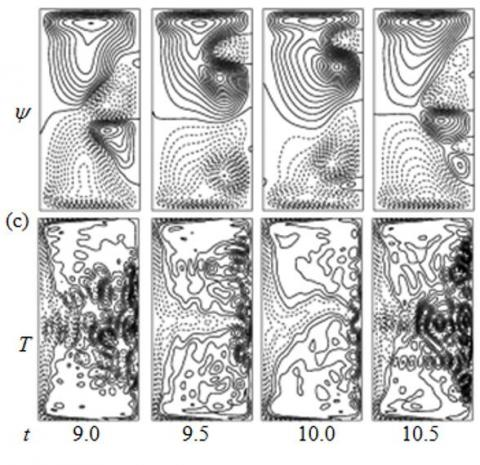Figure 3. (a) Time-progression of $\lambda$, (b) Phase plot, (c) Seconary flow (top) and isotherm (bottom); for Tr = 100

## 4a.jpg## 4c.jpg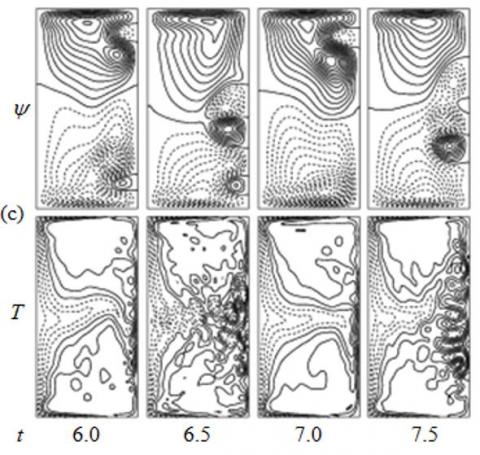Figure 4. (a) Time-progression of λ, (b) Phase plot, (c) Seconary flow (top) and isotherm (bottom); for Tr = 250

## 5a.jpg## 5c.jpgFigure 5. (a) Time-progression of λ, (b) Phase plot, (c) Seconary flow (top) and isotherm (bottom); for Tr = 300

## 6a.jpg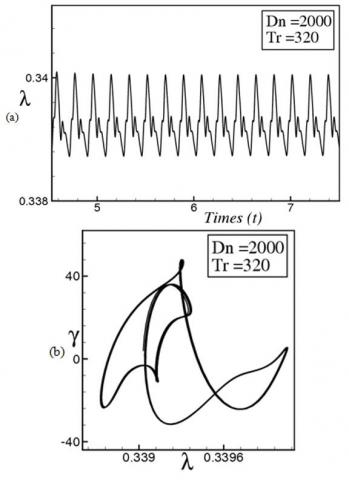## 6c.jpg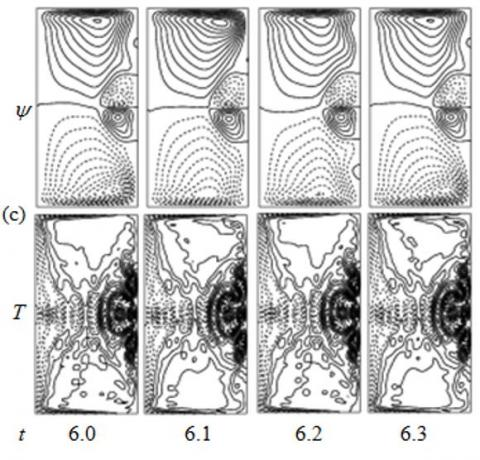Figure 6. (a) Time-progression of λ, (b) Phase plot, (c) Seconary flow (top) and isotherm (bottom); for Tr = 320

## 7a.jpg## 7c.jpgFigure 7. (a) Time-progression of λ, (b) Phase plot, (c) Seconary flow (top) and isotherm (bottom); for Tr = 350

## 8.jpg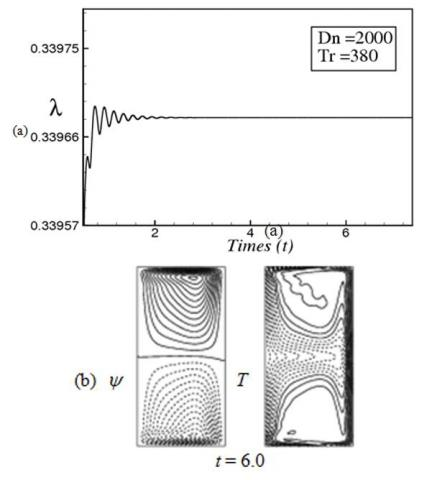Figure 8. (a) Time-progression of λ, (b) Seconary flow (top) and isotherm (bottom); for Tr = 380

## 9.jpg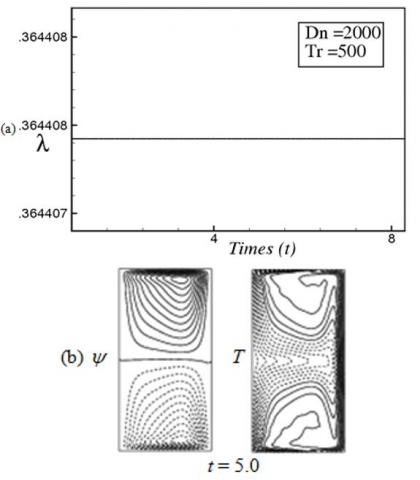Figure 9. (a) Time-progression of λ, (b) Seconary flow (top) and isotherm (bottom); for Tr = 500

If Tr is increased further in the positive direction, we obtain steady-state solution as we predict the general scenario of fluid dynamics. Figures 8(a) and 9(a) show time evolution results for Tr = 380 and Tr = 500 respectively, and it is found that the unsteady flow is a steady-state solution for both the cases. Since the flow is steady-state, a single contour of fluid velocity and isotherm is shown in Figures 8(b) and 9(b) for Tr = 380 and Tr = 500 respectively, and it is found that the time-dependent flows are asymmetric 2-vortex solutions for both the cases. The present study shows that temperature distribution is consistent with the secondary vortices, and secondary flow enhances heat transfer in the flow through vortex generation. In this study, it is found that combined action of the centrifugal, Coriolis and buoyancy forces aids to increase the number of secondary vortices, sometimes called Dean vortices, and as the flow becomes chaotic, the number of secondary vortices increases and consequently heat transfer is enhanced significantly from the heated wall to the fluid. In this regard, it should be noted that, the occurrence of the chaotic state, as presented in the present study, is related with destabilization of the periodic or quasi-periodic solutions which reminds us the case of Lorenz attractor . It may be possible that the transition in the present study is caused by a similar mechanism as that of Ruelle-Takens scenario  in the laminar flow.

4.2 Vortex structure for positive rotation

In order to see the vortex-structure of secondary flows for positive rotation, streamlines of secondary flow and isotherms are predicted for the steady-state, periodic/multi-periodic and chaotic solutions for various values of Tr as shown in Figure 10. Figure 10 shows velocity contours and isotherm for the chaotic solutions at Tr = 0, 100 and 250, periodic or multi-periodic solutions at Tr = 300, 350 and 370, and steady-state solutions at Tr = 500 and 1000, where 4- to 6-vortex solutions are obtained for the chaotic solution, 4-vortex for the periodic/multi-periodic solution while only 2-vortex for the steady-state solution. It is found that the number of secondary vortices increases as the flow becomes chaotic, and therefore it is suggested that chaotic solutions enhance heat transfer more efficiently than the steady-state or periodic solutions; this is because many secondary vortices are engendered at the outer concave wall of the duct  if the flow is chaotic. The periodic and chaotic behaviors are well discussed by Mondal et al.  and Mondal et al. . Later, steady and unsteady behavior together with the flow velocity and the isotherms for the rotating and non-rotating curved duct is investigated by Nahid et al. , Nipa et al. , and Dolon et al.  study for square and rectangular rotating and non-rotating duct. They showed that though the unsteady behavior shows almost the same scenario such as steady-state, periodic, multi-periodic, and chaotic the flow characteristics are certainly influenced by the size, curvature, rotation of the duct. The irregular oscillations at Tr = 0, 100 or 250 are termed as strong chaos . The study shows that the number of secondary vortices is significantly increases for the chaotic solution at small rotation; however, as the rotation is increased gradually in the positive direction the chaotic flow turns into steady-state flow via multi-periodic and periodic flows, and consequently the number of secondary vortices decreases. Temperature profiles show that they are compatible with the secondary vortices, and a strong interaction is observed between the secondary flow pattern and temperature distribution.

## 10.jpgFigure 10. Vortex structure of secondary flows and isotherm for various values of Tr

4.3 Case II: Negative rotation

Now, we performed time-history analysis for the negative rotation of the duct at Dn = 2000 and $\delta=0.5$. Figure 11(a) shows time progression of λ for Tr = -100. It is found that the unsteady flow at Tr = -100 is a chaotic oscillation, which is accurately justified by drawing the phase space as shown in Figure 11(b). As seen in Figure 11(b), the flow creates irregular orbits covering the $\lambda-\gamma$ plane so that the flow displayed in Figure 11(a) is chaotic at Tr = -100. To observe the pattern variation of velocity profiles and isotherm, streamlines of secondary flow and isotherm are obtained as shown in Figure 11(c) for Tr = -100, where it is found that the chaotic oscillation at Tr = -100 is an asymmetric 4-vortex solution. Here we also observe that the streamlines of temperature profiles are consistence with the secondary vortices and convective heat generation is stronger in the whole position of the contour.

## 11a.jpg## 11c.jpgFigure 11. (a) Time-progression of λ, (b) Phase plot, (c) Seconary flow (top) and isotherm (bottom); for Tr = - 100

## 12a.jpg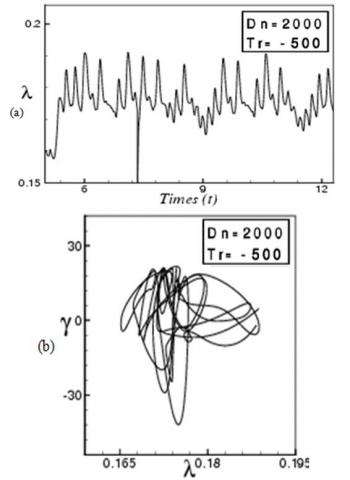## 12c.jpgFigure 12. (a) Time-progression of λ, (b) Phase plot, (c) Seconary flow (top) and isotherm (bottom); for Tr = - 500

## 13a.jpg## 13c.jpg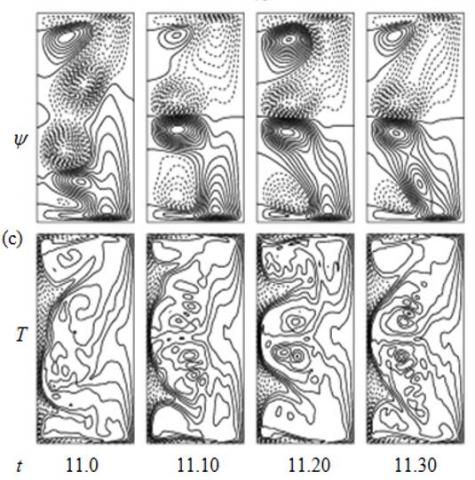Figure 13. (a) Time-progression of λ, (b) Phase plot, (c) Seconary flow (top) and isotherm (bottom); for Tr = - 700

## 14a.jpg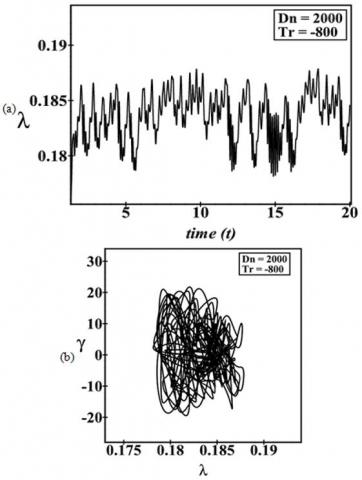## 14c.jpgFigure 14. (a) Time-progression of λ, (b) Phase plot, (c) Seconary flow (top) and isotherm (bottom); for Tr = -800

## 15a.jpg## 15c.jpg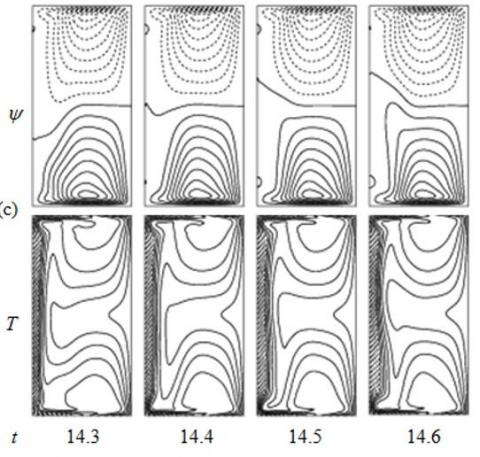Figure 15. (a) Time-progression of λ, (b) Phase plot, (c) Seconary flow (top) and isotherm (bottom); for Tr = -850

We continue this process and perform time dependent solution of λ for Tr = -500 and Tr = -700 as shown in Figure 12(a) and 13(a), respectively. As seen in Figures 12(a) and 13(a), the time-dependent solutions at Tr = -500 and Tr = -700 are also chaotic. To observe the mode of oscillation of the chaotic oscillation, we draw the phase spaces of the time change of l for Tr = -500 and Tr = -700 as shown in Figures 12(b) and 13(b) respectively, and it is found that the transient solutions at Tr = -500 and -700 are weak chaotic solution. Typical contours of secondary velocity and isotherms for Tr = -500 and Tr = -700 are shown in Figures 12(c) and 13(c) respectively, where it is found that the chaotic oscillation at Tr = -500 and Tr = -700 are asymmetric 4- to 6-vortex solution. Temperature profiles show that the streamlines of heat flow is uniformly distributed to all parts of the contour transferring heat from outer wall to the fluid, and the combined effects of rotation, pressure and buoyancy significantly change and increase the number of secondary vortices which play an important role in transferring heat from the heated wall to the fluid. Time advancement of λ at Tr = -800 is shown in Figure 14(a), which shows that the unsteady flow at Tr = -800 is chaotic. This chaotic oscillation is well verified by drawing the phase space of the time advancement of l as shown in Figure 14(b). It is seen that the orbits of the flow-evolution intersect robustly which does not move in a regular pattern so that that the flow is chaotic at Tr = -800. To see the pattern variation of velocity profiles, typical contours of secondary flow and isotherm for Tr = -800 are attained as presented in Figure 14(c), and it is found that the chaotic flow oscillates in the asymmetric 4-vortex solution. If we accelerate the rotational speed in the negative direction at Tr = -850, time progression result shows that the unsteady flow passes a regular pattern for a certain time interval that means the flow is periodic at Tr  = -850 as shown in Figure 15(a). This periodic oscillation is well confirmed by drawing the phase space as shown in Figure 15(b). From Figure 15(b), it is visualized that the orbit of the flow-evolution does not intersect rather it moves in a regular pattern creating a single orbit, which signifies that the flow is time-periodic. Contours secondary velocity and isotherm for Tr = -850 are then obtained as presented in Figure 15(c), and it is found that they oscillate between asymmetric 2-vortex solution. If we increase the simulation for strongly negative rotation of the duct, for example, Tr = -1000 as shown in Figure 16(a), it is found that the periodic flow is changed into a steady-state nature, which creates asymmetric 2-vortex solution as shown in Figure 16(b). In this regard, it should be noted that Mondal et al.  executed spectral numerical study on unsteady fluid flow through a curved rectangular duct of small aspect ratio over a wide range of the Dean number (Dn) and the Grashof number (Gr). They obtained maximum 4-vortex solution and mentioned that the unsteady flow becomes steady-state, periodic, multi-periodic or chaotic if Dn or Gr is increased. for in the ongoing study, the general scenario of the fluid dynamics which is- ‘Chaotic→multi-periodic→periodic→steady-state’ is confirmed for both positive and negative rotation of the duct.

## 16.jpg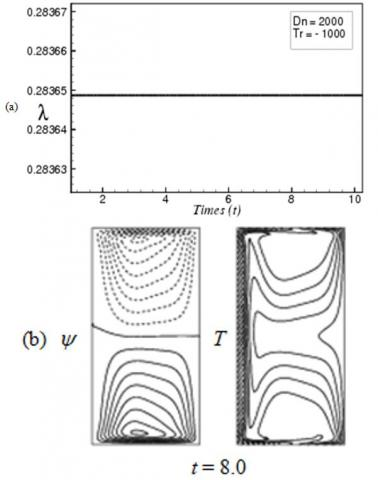Figure 16. (a) Time-progression of λ, (b) Seconary flow (top) and isotherm (bottom); for Tr = -1000

4.4 Bar diagram of secondary flow structure

Here, to observe the vortex-structure of secondary flows for the time-dependent solution at a glance, we draw a bar diagram to represent the formation of secondary vortices for time-dependent solutions in the Taylor number vs. number of vortices (Tr-θ) plane for both positive and negative rotation of the duct. Figure 17 shows vortex-structure of secondary flows on unsteady solutions for δ=0.5. As seen in Figure 17, two- to multi-vortex solutions are produced at the same value of Tr. It is found that maximum 8-vortex solution is attained at Tr = 0. However, as the rotation is imposed either in the positive or in the negative direction, for example when Tr = 1000 or Tr = -1000, the number of secondary vortices declines gradually and finally we get only 2-vortex solution. The reason is that strong centrifugal force and Coriolis force act in an opposite manner where buoyancy force is predominate and consequently number of secondary vortices decreases.

## 17.jpgFigure 17. Vortex structure of secondary flows for various values of Tr at δ=0.5 and Dn = 2000

4.5 Convective heat transfer

The Nusselt number Nu, defined in Eq. (15), can be used as an index of horizontal heat transfer from the walls to the fluid. If the flow field is not steady, time-average of the Nusselt number, $N u_{\tau}$ is used as defined in Eq. (16). To study the convective heat transfer, variation of the steady values of Nu with Tr is shown in Figure 18 for both positive and negative rotation. Time-average of Nu, calculated by the time-evolution computations on the inner and outer sidewalls for different solutions is also shown in the same figure. As seen in Figure 18(a) for positive rotation, Nu is larger at the heated wall than that at the cooling wall. It is also observed that Nu declines as Tr rises, and Nu for the cooling wall declines rapidly than the Nu for the heated wall. The reason is that the flow is chaotic at small Tr and as Tr increases the flow becomes steady-state. It is very interesting that, as seen in Figure 18(b) for the negative rotation, Nu for the heated wall increases rapidly for the heated wall while drops rapidly for the cooling wall as Tr increases in the negative direction. The reason is that, for the case of negative rotation heat transfer occurs substantially from the heated wall to the fluid than the cooling wall due to accumulating the significant number of secondary vortices at the outer concave wall.

## 18.jpg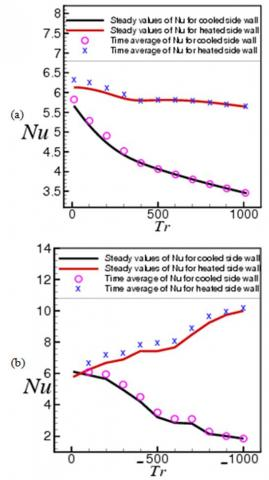Figure 18. Variation of the Nusselt number (Nu) with the Taylor number (Tr); (a) Positive rotation, (b) Negative rotation

Then, to study convective heat transfer, temperature gradients (TG) on the cooling and heated sidewalls are calculated as shown in Figure 19 for positive rotation and in Figure 20 for negative rotation. As seen in Figures 19(a) and 20(a), $\frac{\partial T}{\partial x}$ on the cooling wall declines in the central region near y=0 as Tr increases. In the same figure, it is also seen that $\frac{\partial T}{\partial x}$ soar up in the regions other than the central region. This is due to advection of the secondary flow in the inward direction, which is a reverse flow of the outward secondary flow in the central region. As seen in Figures 19(b) and 20(b), $\frac{\partial T}{\partial x}$ raises monotonically over the whole region as Tr goes up from zero. It is observed that $\frac{\partial T}{\partial x}$ raises much higher at Tr = 0 or small values of Tr than that at large Tr. Thus, as seen in Figures 19 and 20, the tendency of increasing TG at the heated wall is much significant than that in the cooling wall, which can be explained by the statement that heat transfer occurs considerably from the heated wall to the fluid and particularly at small Tr where the flow is chaotic.

## 19.jpg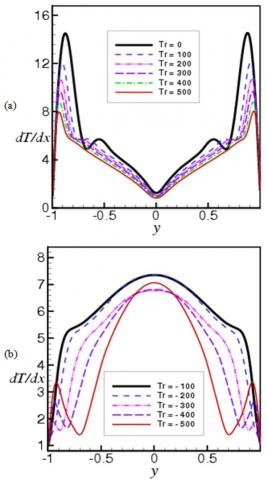Figure 19. Temperature gradients for positive rotation; (a) cooled wall, (b) heated wall

## 20.jpgFigure 20. Temperature gradients for negative rotation; (a) cooled wall, (b) heated wall

4.6 Validation of the numerical result

Here, we represent the validation of our computational results with the experimental studies performed by some authors. Figure 21 shows a comparative study of our computational result with the experimental investigation obtained by Chandratilleke  for the flow through a curved rectangular duct of aspect ratio 2. We see that our computational results have a good agreement with the experimental data. Note that, till now no experimental studies have been found for rotating curved rectangular duct flow.

## 21.jpg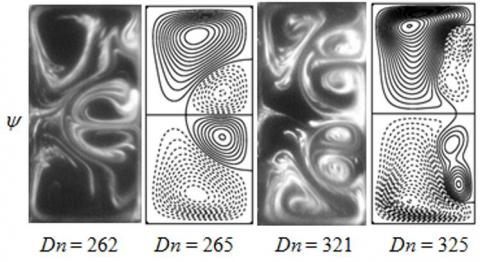Figure 21. Experimental vs. numerical results. Left: experimental result by Chandratilleke  and right: numerical result by the authors

5. Concluding Remarks

Hydro-thermal behavior of transient fluid flow with natural and forced convective heat transfer through a rotating curved rectangular duct of aspect ratio 2 and curvature ratio 0.5 is investigated numerically with the aid of spectral technique considering a strong pressure gradient force in the axial direction. The outer wall of the duct is heated while the inner wall cooled, the top and bottom walls being thermally insulated. The system is rotated about the vertical axis in the positive and negative direction over a wide range of the Taylor number (Tr). The numerical results are validated with the experimental data and a good matching is observed. The following conclusions have been drawn from the present study;

• Time-history analysis as well as phase-space of the time progression results shows that the transient flow undergoes in the consequence ‘chaotic→ multi-periodic→periodic→ steady-state’, if Tr is increased either in the positive or in the negative direction.
• Flow regimes are identified for different types of physically realizable solutions which shows that for positive rotation, the transient flow is chaotic for 0≤Tr≤250, multi-periodic or periodic for 300≤Tr≤370and steady-state for 380≤Tr≤1000; for negative rotation, however, the transient flow is chaotic for -700≤Tr<0, periodic/multi-periodic for -900≤Tr≤-750 and steady-state for -1000≤Tr≤-900.
• Vortex generation of secondary flows show that the transient flow is asymmetric 2- to 8-vortex for the chaotic solution, 4- to 6-vortex for the periodic/multi-periodic solution while only 2-vortex for the steady-state solution. The number of secondary vortices is significantly higher for the chaotic solution, while few for the steady-state solution.
• To study convective heat transfer, it is found that for positive rotation, Nusselt numbers (Nu) declines as Tr rises, and Nu for the cooling wall drops rapidly than the Nu for the heated wall. For the negative rotation, however, Nu for the heated wall upsurges rapidly for the heated wall but falls promptly for the cooling wall.
• The study shows that chaotic solutions boost heat transfer more effectively than the steady-state or other solutions due to significant number of Dean vortices at the outer concave wall. A strong interaction is observed between the heating-induced buoyancy force and centrifugal-Coriolis instability in the rotating curved passage that inspires fluid mixing and subsequently enhances heat transfer in the fluid at a great deal.
Nomenclature
 Dn Dean number Gr Grashof number Pr Prandtl number A Aspect ratio L Radius of the curvature X Horizontal axis Y Vertical axis Z Axis in the direction of the main flow U Velocity components in the x-direction V Velocity components in the y-direction W Velocity components in the z-direction T Temperature T Time Greek symbols δ Curvature of the duct ρ Density λ Resistance coefficient μ Viscosity k Thermal diffusivity v Kinematic viscosity ψ Sectional stream function
References

 Dean, W.R., Hurst, J.M. (1959). Note on the motion of fluid in a curved pipe. Mathematika, 6(1): 77-85. https://doi.org/10.1112/S0025579300001947

 Berger, S.A., Talbot, L., Yao, L.S. (1983). Flow in curved pipes. Annual Review of Fluid Mechanics, 15(1): 461-512. https://doi.org/10.1146/annurev.fl.15.010183.002333

 Nandakumar, K., Masliyah, J.H. (1986). Swirling flow and heat transfer in coiled and twisted pipes. Advanced Transport Process, 4: 49-112.

 Ito, H. (1987). Flow in curved pipes. JSME International Journal, 30(262): 543-552. https://doi.org/10.1299/jsme1987.30.543

 Yanase, S., Kaga, Y., Daikai, R. (2002). Laminar flow through a curved rectangular duct over a wide range of aspect ratio. Fluid Dynamics Research, 31(3): 151-183. https://doi.org/10.1016/S0169-5983(02)00103-X

 Mondal, R.N. (2006). Isothermal and non-isothermal flows through curved ducts with square and rectangular cross sections. Mechanical Engineering.

 Chandratilleke, T.T., Nursubyakto. (2003). Numerical prediction of secondary flow and convective heat transfer in externally heated curved rectangular ducts. International Journal of Thermal Science, 42(2): 187-198. https://doi.org/10.1016/S1290-0729(02)00018-2

 Ghia, K.N., Sokhey, J.S. (1977). Laminar incompressible viscous flow in curved ducts of rectangular cross-section. Trans. ASME I: Journal of Fluids Engineering, 99(4): 640-648. https://doi.org/10.1115/1.3448875

 Ishigaki, H. (1996). Laminar flow in rotating curved pipes. Journal of Fluid Mechanics, 329: 373-388. https://doi.org/10.1017/S0022112096008956

 Selmi, M., Nandakumar, K., Finlay, W.H. (1994). A bifurcation study of viscous flow through a rotating curved duct. Journal of Fluid Mechanics, 262: 353-375. https://doi.org/10.1017/S0022112094000534

 Wang, L.Q., Cheng, K.C. (1996). Flow transitions and combined free and forced convective heat transfer in rotating curved channels: The case of positive rotation. Physics of Fluids, 8(6): 1553-1573. https://doi.org/10.1063/1.868930

 Mondal, R.N., Watanabe, T., Hossain, M.A., Yanase, S. (2017). Vortex-structure and unsteady solutions with convective heat transfer trough a curved duct. Journal of Thermophysics and Heat Transfer, 31(1): 243-253. https://doi.org/10.2514/1.T4913

 Chandratilleke, T.T., Nadim, N., Narayanaswamy, R. (2012). Vortex structure-based analysis of laminar flow behavior and thermal characteristics in curved ducts. International Journal of Thermal Science, 59: 75-86. https://doi.org/10.1016/j.ijthermalsci.2012.04.014

 Norouzi, M., Kayhani, M.H., Nobari, M.R.H., Demneh, M.K. (2009). Convective heat transfer of viscoelastic flow in a curved duct. World Academy of Science, Engineering and Technology, 32: 327-333. https://doi.org/10.5281/zenodo.1081071

 Chandratilleke, T.T., Nadim, N., Narayanaswamy, R. (2013). Analysis of secondary flow instability and forced convection in fluid flow through rectangular and elliptical curved ducts. Heat Transfer Engineering, 34(14): 1237-1248. https://doi.org/10.1080/01457632.2013.777249

 Hasan, M.S., Mondal, R.N., Kouchi, T., Yanase, S. (2019). Hydrodynamic instability with convective heat transfer through a curved channel with strong rotational speed. AIP Conference Proceedings, 2121(1): 030006. https://doi.org/10.1063/1.5115851

 Hasan, M.S., Islam, M.M., Ray, S.C., Mondal, R.N. (2019). Bifurcation structure and unsteady solutions through a curved square duct with bottom wall heating and cooling from the ceiling. AIP Conference Proceedings, 2121(1): 050003. https://doi.org/10.1063/1.5115890

 Yanase, S., Nishiyama, K. (1988). On the bifurcation of laminar flows through a curved rectangular tube. Journal of Physical Society of Japan, 57(11): 3790-3795. https://doi.org/10.1143/JPSJ.57.3790

 Wang, L., Yang, T. (2005). Periodic oscillation in curved duct flows. Physica D, 200(3-4): 296-302. https://doi.org/10.1016/j.physd.2004.11.003

 Mondal, R.N., Kaga, Y., Hyakutake, T., Yanase, S. (2007). Bifurcation diagram for two- dimensional steady flow and unsteady solutions in a curved square duct. Fluid Dynamics Research, 39(5): 413-446. https://doi.org/10.1016/j.fluiddyn.2006.10.001

 Yamamoto, K., Xiaoyun, W., Kazuo, N., Yasutaka, H. (2006). Visualization of Taylor-Dean Flow in a curved duct of square cross-section. Fluid Dynamics Research, 38(1): 1-18. https://doi.org/10.1016/j.fluiddyn.2005.09.002

 Mondal, R.N., Kaga, Y., Hyakutake, T., Yanase, S. (2006). Effects of curvature and convective heat transfer in curved square duct flows. Trans. ASME, Journal of Fluids Engineering, 128(9): 1013-1022. https://doi.org/10.1115/1.2236131

 Mondal, R.N., Ray, S.C., Yanase, S. (2014). Combined effects of centrifugal and coriolis instability of the flow through a rotating curved duct with rectangular cross section. Open Journal of Fluid Dynamics, 4(1): 1-14. https://doi.org/10.4236/ojfd.2014.41001

 Razavi, S.E., Soltanipour, H., Choupani, P. (2015). Second law analysis of laminar forced convection in a rotating curved duct. Thermal Sciences, 19(1): 95-107. https://doi.org/10.2298/TSCI120606034R

 Krishna, C.V., Gundiah, N., Arakeri, J.H. (2017). Separation and secondary structures due to unsteady flow in a curved pipe. Journal of Fluid Mechanics, 815: 25-59. https://doi.org/10.1017/jfm.2017.7

 Islam, M.Z., Mondal, R.N., Rashidi, M.M. (2017). Dean-Taylor flow with convective heat transfer through a coiled duct. Computers and Fluids, 149: 41-55. https://doi.org/10.1016/j.compfluid.2017.03.001

 Hasan, M.S., Mondal, R.N., Lorenzini, G. (2019). Numerical Prediction of non-isothermal flow with convective heat transfer through a rotating curved square channel with bottom wall heating and cooling from the ceiling. International Journal of Heat and Technology, 37(3): 710-726. https://doi.org/10.18280/ijht.370307

 Hasan, M.S., Islam, M.S., Badsha, M.F., Mondal, R.N., Lorenzini, G. (2020). Numerical investigation on the transition of fluid flow characteristics through a rotating curved duct. International Journal of Applied Mechanics and Engineering, 25(3): 45-63. https://doi.org/10.2478/ijame-2020-0034

 Hasan, M.S., Mondal, R.N., Lorenzini, G. (2020). Coriolis force effect in steady and unsteady flow characteristics with convective heat transfer through a curved square duct. International Journal of Mechanical Engineering, 5(1): 1-39.

 Chandratilleke, T.T. (2001). Secondary flow characteristics and convective heat transfer in a curved rectangular duct with external heating. 5th World Conference on Experimental Heat Transfer, Fluid Mechanics and Thermodynamics, Thessaloniki, Greece.

 Yanase, S. Mondal, R.N., Kaga, Y. (2005). Numerical study of non-isothermal flow with convective heat transfer in a curved rectangular duct. International Journal of Thermal Science, 44(11): 1047-1060. https://doi.org/10.1016/j.ijthermalsci.2005.03.013

 Norouzi, M., Kayhani, M.H., Nobari, M.R.H., Demneh, M.K. (2009). Convective heat transfer of viscoelastic flow in a curved duct. World Academy of Science, Engineering and Technology, 32: 327-333. https://doi.org/10.5281/zenodo.1081071

 Norouzi, M., Biglari, N. (2013). An analytical solution for dean flow in curved ducts with rectangular cross section. Physics of Fluids, 25(5): 053602. https://doi.org/10.1063/1.4803556

 Wu, X.Y., Lai, S.D., Yamamoto, K., Yanase, S. (2013). Numerical analysis of the flow in a curved duct. Advanced Materials Research, 706-708: 1450-1453. https://doi.org/10.4028/www.scientific.net/AMR.706-708.1450

 Hasan, M.S., Mondal, R.N., Lorenzini, G. (2019). Centrifugal instability with convective heat transfer through a tightly coiled square duct. Mathematical Modelling of Engineering Problems, 6(3): 397-408. https://doi.org/10.18280/mmep.060311

 Hasan, M.S., Mondal, R.N., Lorenzini, G. (2020). Physics of bifurcation of the flow and heat transfer through a curved duct with natural and forced convection. Chinese Journal of Physics, 67: 428-457. https://doi.org/10.1016/j.cjph.2020.07.004

 Ray, S.C., Hasan, M.S., Mondal, R.N. (2020). On the onset of hydrodynamic instability with convective heat transfer through a rotating curved rectangular duct. Mathematical Modelling of Engineering Problems, 7(1): 31-44. https://doi.org/10.18280/mmep.070105

 Gottlieb, D., Orazag, S.A. (1977). Numerical analysis of spectral methods: Theory and applications. Society for Industrial and Applied Mathematics, Philadelphia. https://doi.org/10.1137/1.9781611970425

 Yanase, S., Watanabe, T., Hyakutake, T. (2008). Traveling-wave solutions of the flow in a curved-square duct. Physics of Fluids, 20(124101): 1-8. https://doi.org/10.1063/1.3029703

 Lorenz, E.N. (1963). Deterministic nonperiodic flow. Journal of the Atmospheric Sciences, 20(2): 130-141. https://doi.org/10.1175/1520-0469(1963)020<0130:DNF>2.0.CO;2

 Ruelle, D. Takens, F. (1971). On the nature of turbulence. Communication in Mathematical Physics, 20: 167-192. https://doi.org/10.1007/BF01646553

 Mondal, R.N., Islam, M.S., Uddin, M.K., Hossain, M.A. (2013). Effects of aspect ratio on unsteady solutions through a curved duct flow. Applied Mathematics and Mechanics, 34(9): 1-16. https://link.springer.com/article/10.1007/s10483-013-1731-8

 Mondal R.N, Islam M.Z, Islam M.S. (2013). Transient heat and fluid flow through a rotating curved rectangular duct: the case of positive and negative rotation. Procedia Engineering, 56: 179-186. https://doi.org/10.1016/j.proeng.2013.03.105

 Islam, M.N., Ray, S.C., Hasan, M.S., Mondal, R.N. (2019). Pressure-driven flow instability with convective heat transfer through a rotating curved rectangular duct with differentially heated top and bottom walls. AIP Conference Proceedings, 2121(1): 030011. https://doi.org/10.1063/1.5115856

 Sultana, M.N., Hasan, M.S., Mondal, R.N. (2019). A numerical study of unsteady heat and fluid flow through a rotating curved channel with variable curvature. AIP Conference Proceedings, 2121(1): 030009. https://doi.org/10.1063/1.5115854

 Dolon, S.N., Hasan, M.S., Ray, S.C., Mondal, R.N. (2019). Vortex-structure of secondary flows with effects of strong curvature on unsteady solutions through a curved rectangular duct of large aspect ratio. AIP Conference Proceedings, 2121(1): 050004. https://doi.org/10.1063/1.5115891

 Mondal, R.N., Islam, M.Z., Islam, M.M., Yanase, S. (2015). Numerical study of unsteady heat and fluid flow through a curved rectangular duct of small aspect ratio. Thammasat International Journal of Science and Technology, 20(4): 1-20. https://ph02.tcithaijo.org/index.php/SciTechAsia/article/view/44164.